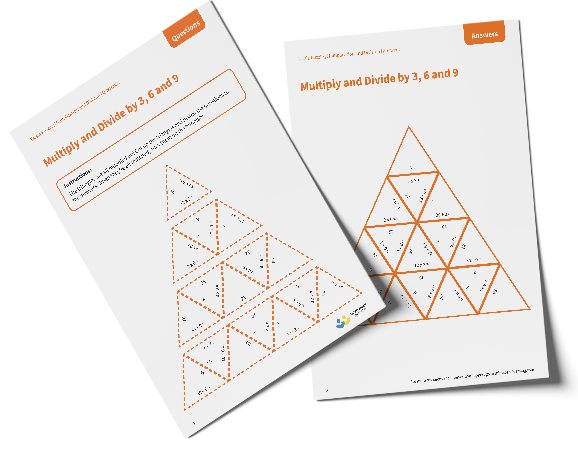Tarsia Puzzle Multiply and Divide by 3, 6 and 9

The triangles in the tarsia puzzle have all been mixed up. In this multiply and divide by 3, 6 and 9 tarsia puzzle, pupils will need to match the multiplication or division question to the correct answer to create one large triangle. This worksheet would be appropriate for Year 4, Year 5 or Year 6.

This multiply and divide by 3, 6 and 9 tarsia puzzle worksheet contains:

• One question sheet
• One template sheet

Year 4, Year 5, Year 6

Multiplication, Division